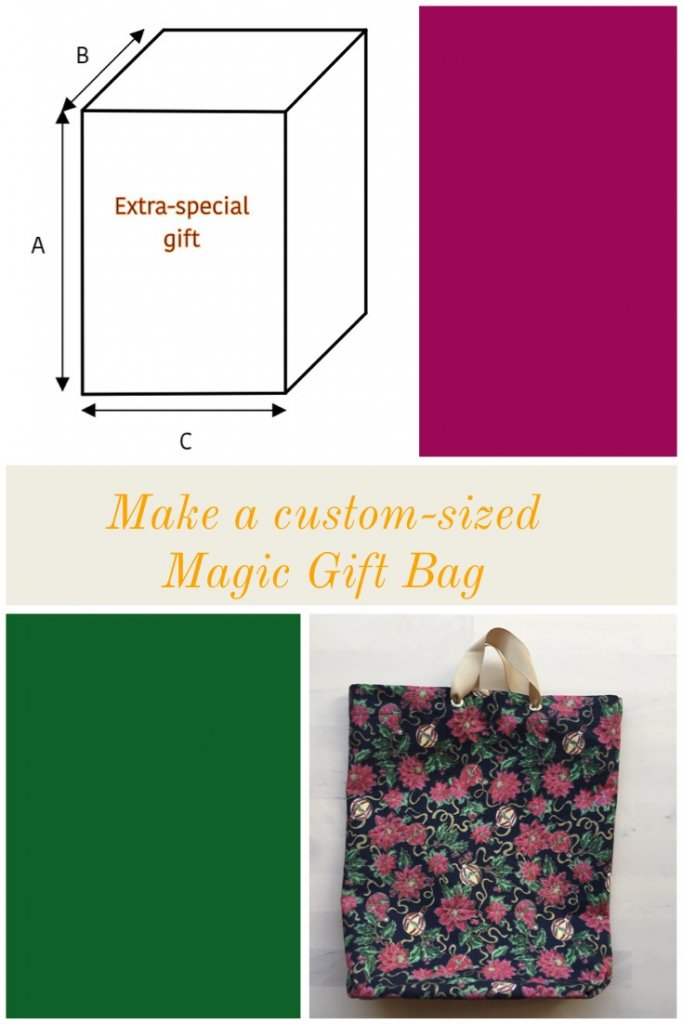# Design a custom-sized gift bag

A few simple measurements and calculations make it easy to create a custom bag for that extra-special gift. You can do it even if you are allergic to math!

You need three measurements: length, depth and width of the gift. Write these down on paper length is called “A”, depth is called “B” and width is called “C”. All measurements are given in inches. If you use centimetres, just convert the added numbers accordingly.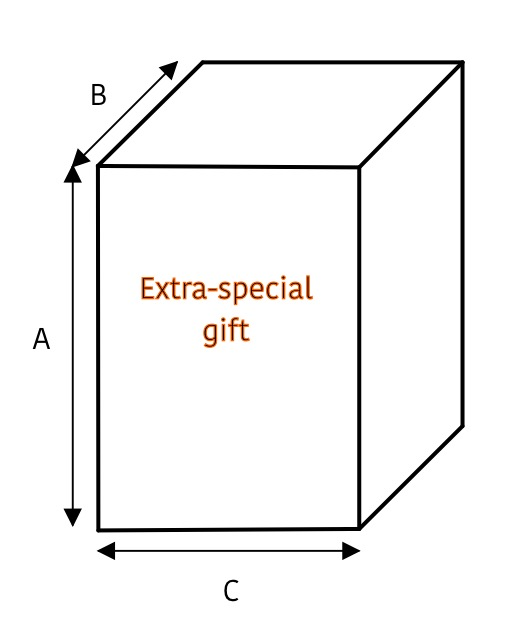To make the magic gift bag, you need three numbers: the length and width of the cut piece and the size of the magic fold. Once you have these, you can use the Magic Gift Bag Tutorial to make any size bag, just use your own numbers instead of the ones given in the tutorial.

#### To calculate length:

Divide the measurement “B” by two, then add “A” and 4

Take this total and multiply by 2

This is the length of the fabric piece.

#### To calculate width:

This is the width of the fabric piece.

#### To calculate the fold:

Divide “B” by two (you already did this for length, so use the same number again)

Add 1/2 to this number, and that is the size of the fold.

Here is an example to show how it works.

This kitchen scale will be my sample gift. It is in a box, so that makes it easier. For soft gifts, you might have to estimate.

The first measurement needed is side A – the height of the box. I want a bag taller than it is wide, so this gift will go in sideways. That makes this the height measurement. A is 10.

If the measurement is an odd fraction, go up to the next closest 1/2″, but don’t go down or it might be too small.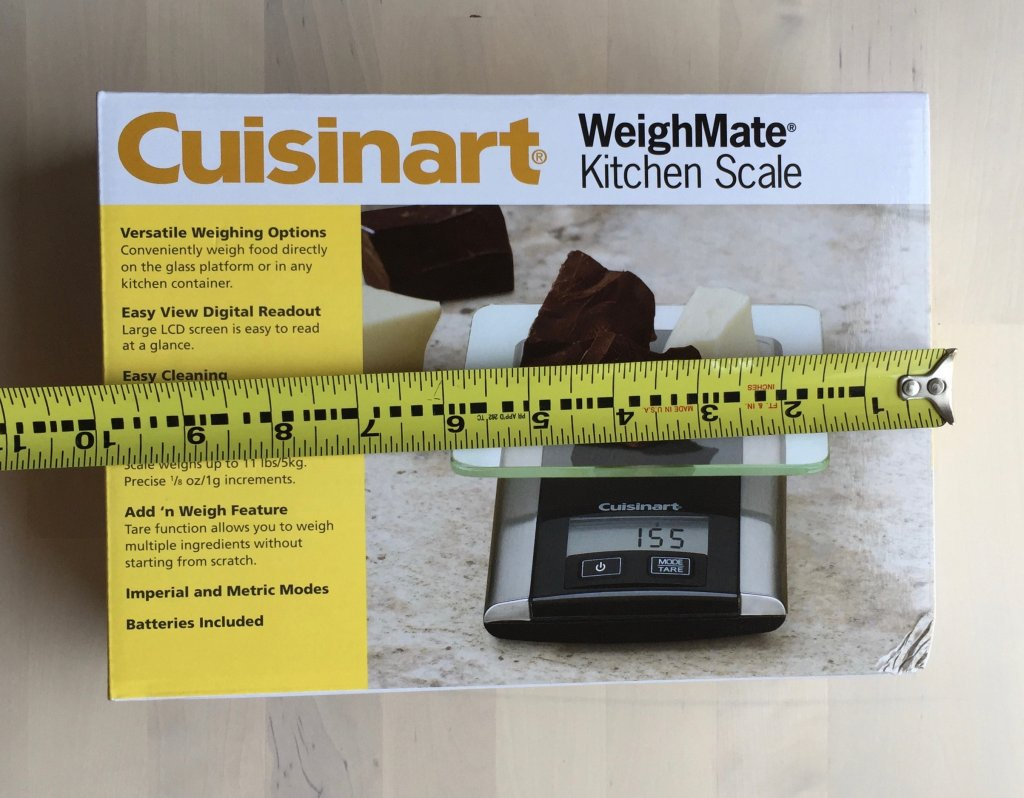The next measurement is the depth of the box. This is the part that will be visible when you look into the top of the bag. B is 3.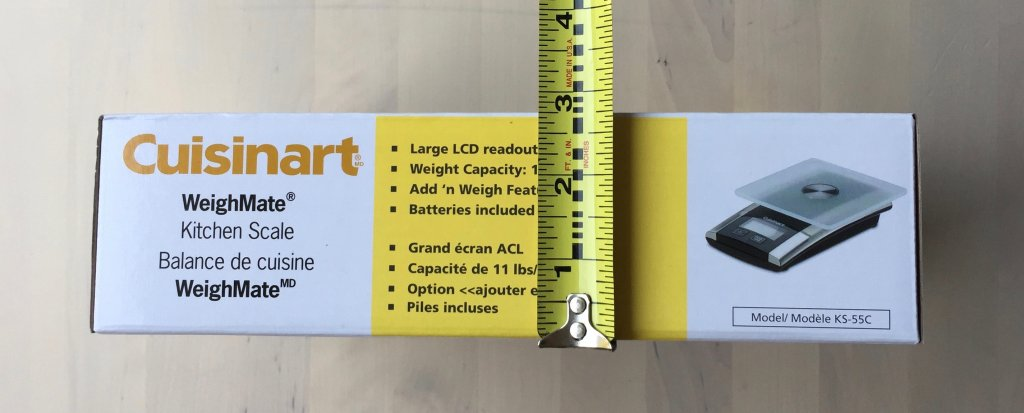Width is the last dimension of the box. C is 7.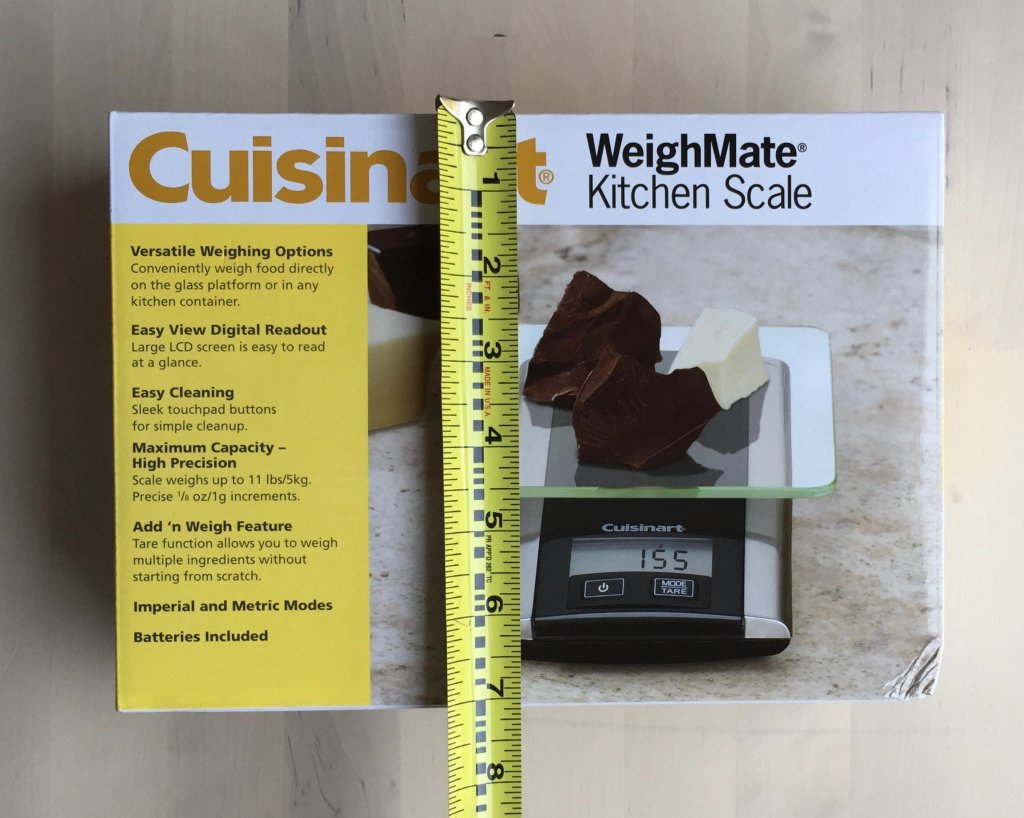Here are the measurements:

A = 10

B = 3

C = 7

length of fabric

B (3) divided by 2 is 1-1/2

1-1/2 + A (10) + 4 is 15-1/2

15-1/2 times 2 is 31

So the fabric piece will be 31″ long

width of fabric

C (7) + B (3) + 2 is 12

So the fabric piece will be 12″ wide

size of fold

B (3) divided by 2 is 1-1/2

1-1/2 + 1/2 is 2

So the size of the fold is 2″